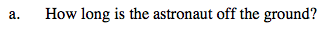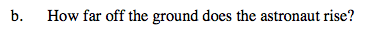### Home > CALC > Chapter 6 > Lesson 6.1.4 > Problem6-48

6-48.$s(t)=\frac{1}{2}at^{2}+v_{0}t+s_{0}$

What do the zeros of s(t) represent?Find the y-value of the vertex of s(t). You can use Algebra or Calculus.